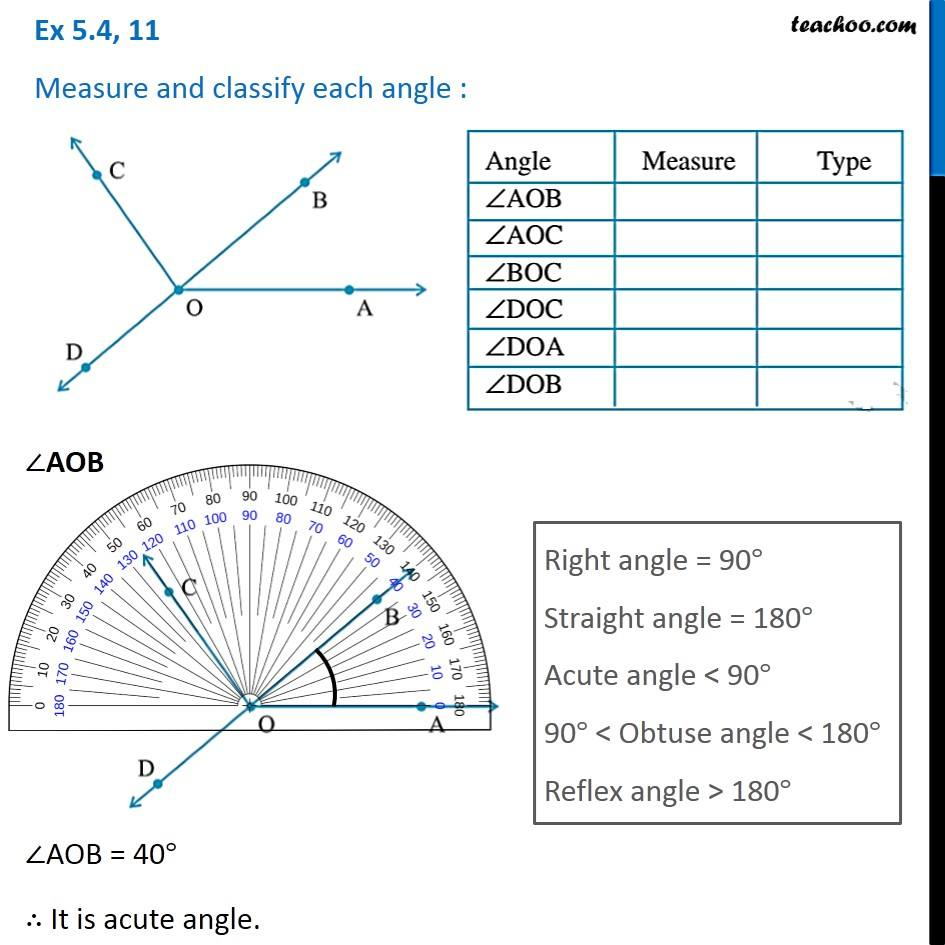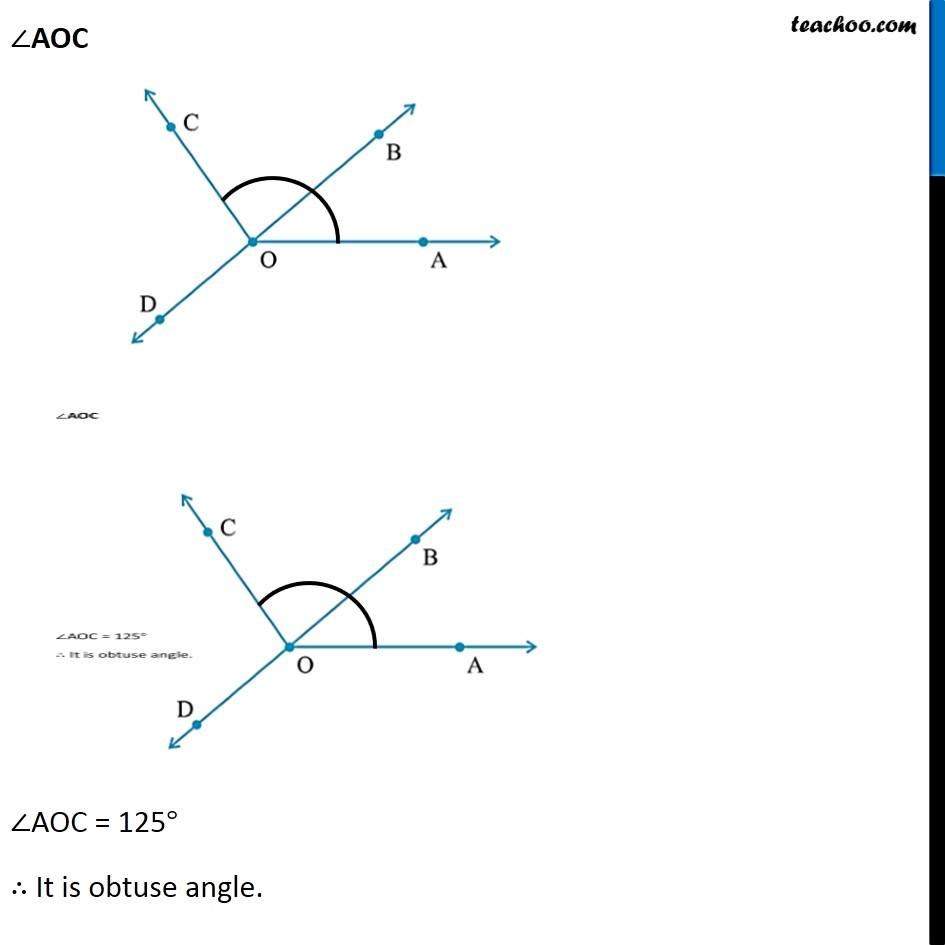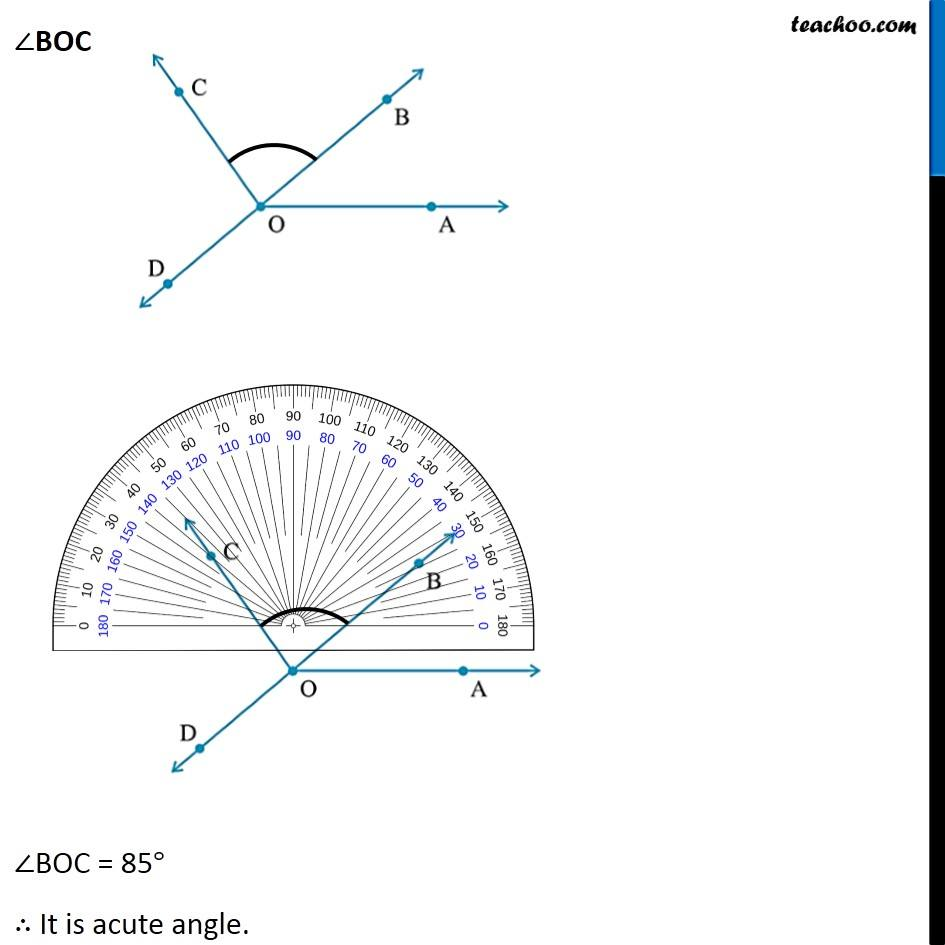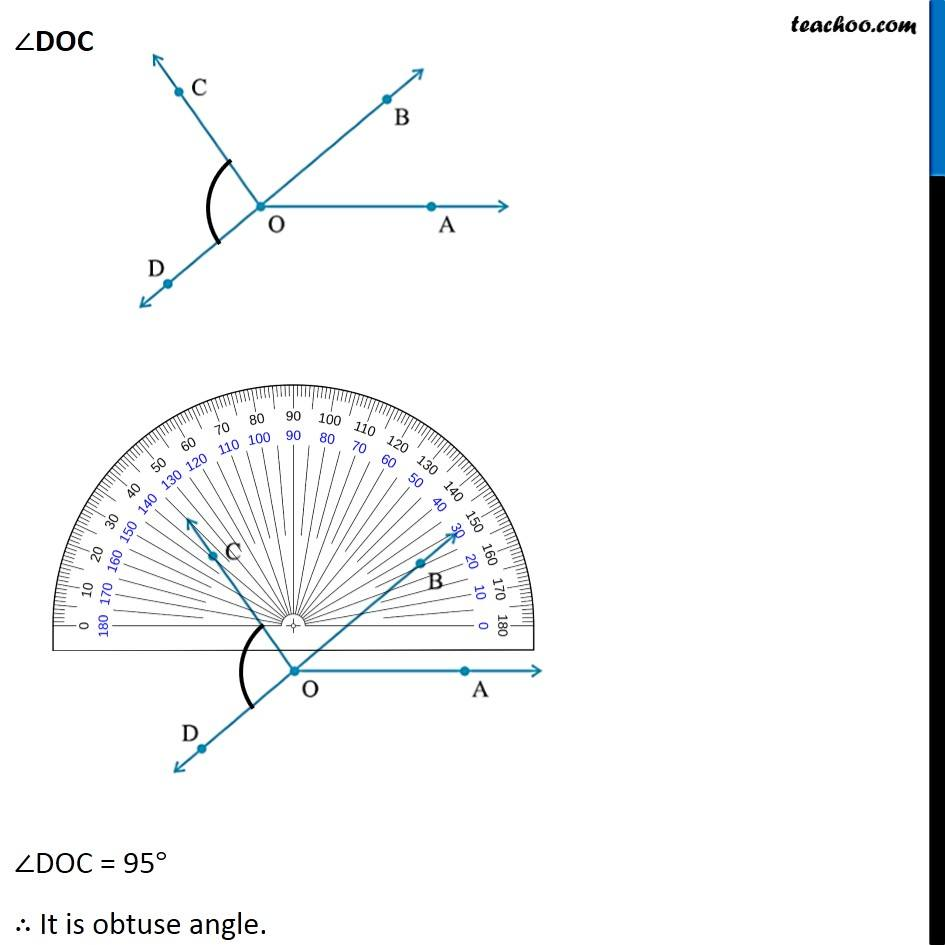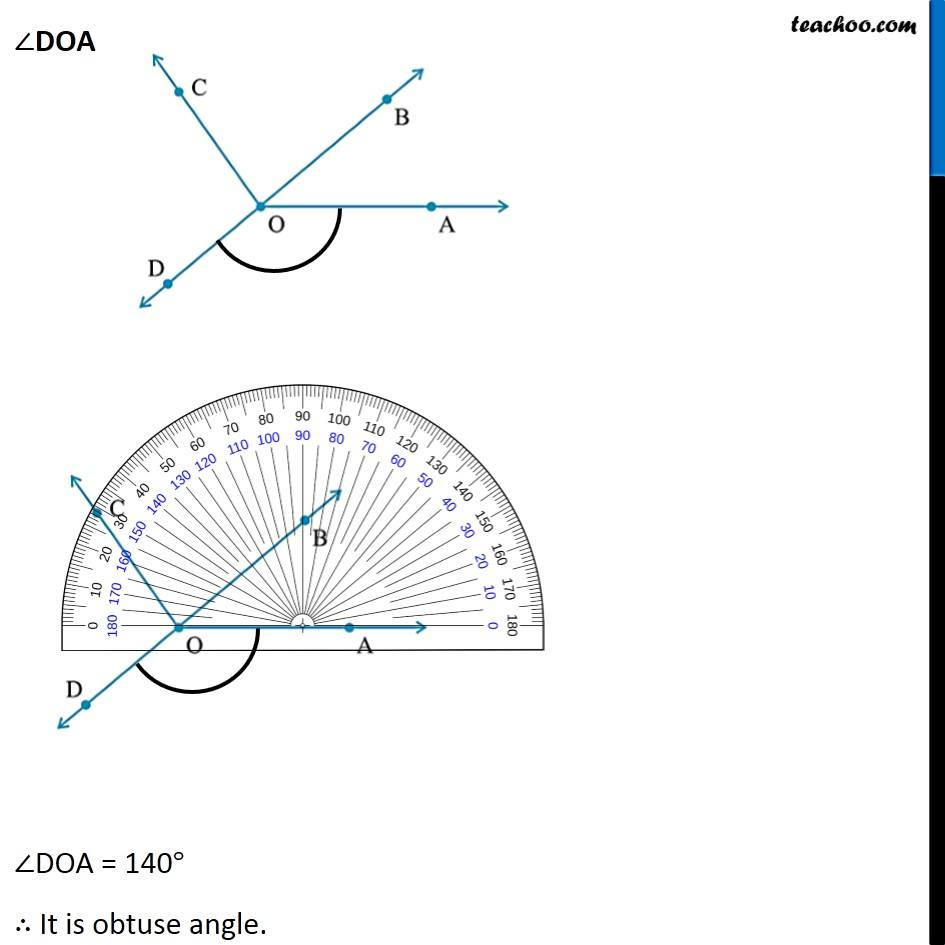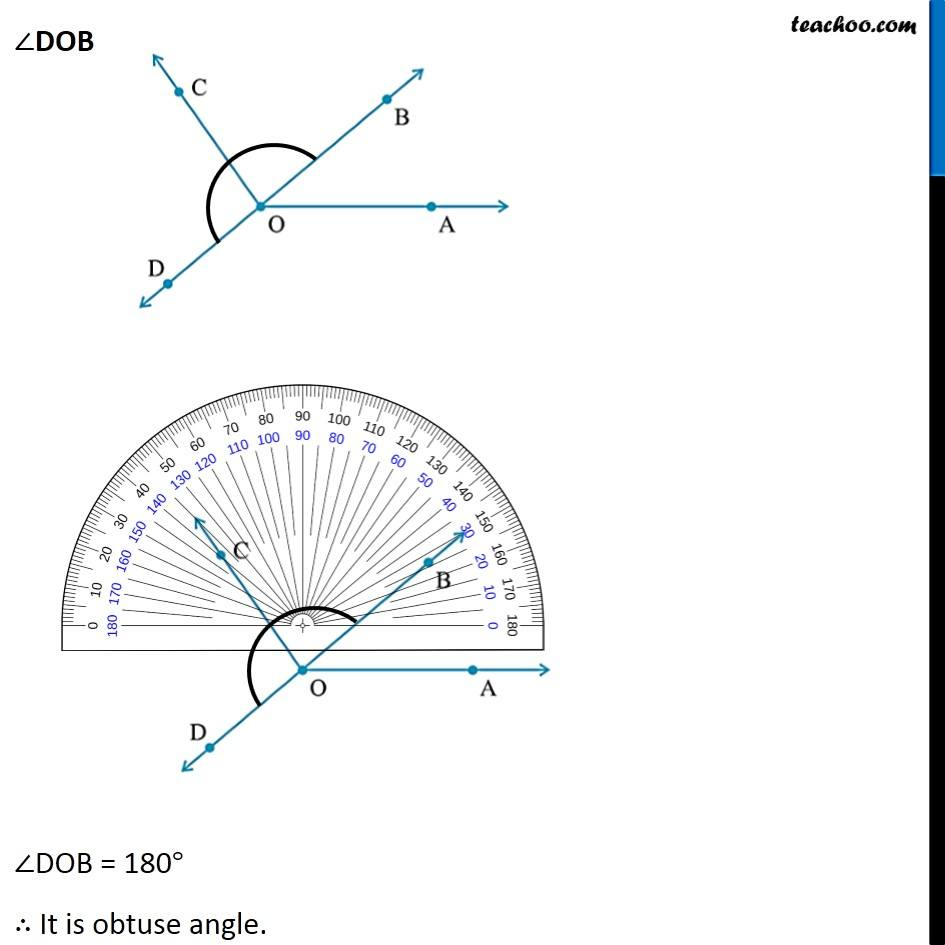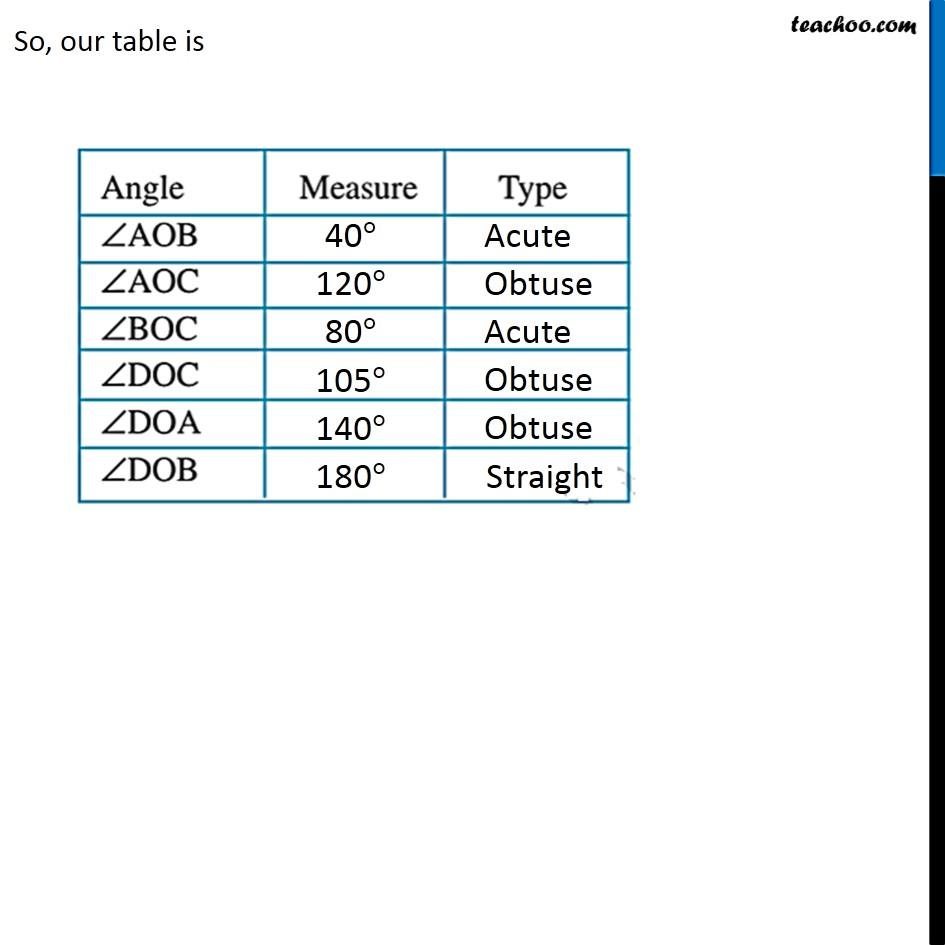Subscribe to our Youtube Channel - https://you.tube/teachoo

1. Chapter 5 Class 6 Understanding Elementary Shapes
2. Concept wise
3. Measuring angles using protractor

Transcript

Ex 5.4, 11 Measure and classify each angle : ∠AOB ∠AOB = 40° ∴ It is acute angle. ∠AOC ∠AOC = 125° ∴ It is obtuse angle. ∠BOC ∠BOC = 85° ∴ It is acute angle. ∠DOC ∠DOC = 95° ∴ It is obtuse angle. ∠DOA ∠DOA = 140° ∴ It is obtuse angle. ∠DOB ∠DOB = 180° ∴ It is obtuse angle. So, our table is

Measuring angles using protractor